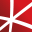# Top 20 NuGet differentiation Packages

The Extreme Optimization Numerical Libraries for .NET are a set of libraries for numerical computing and data analysis. This is the main package that contains all the core functionality. For optimal performance, we strongly recommend also referencing one of the native packages based on Intel's Mat...
Foundational classes for financial, engineering, and scientific applications, including complex number classes, general vector and matrix classes, structured sparse matrix classes and factorizations, general sparse matrix classes and factorizations, general matrix decompositions, least squares solut...
Foundational classes for financial, engineering, and scientific applications, including complex number classes, general vector and matrix classes, structured sparse matrix classes and factorizations, general sparse matrix classes and factorizations, general matrix decompositions, least squares solut...## NMath - Standard Library - Windows - x86 and x64

Foundational classes for financial, engineering, and scientific applications, including complex number classes, general vector and matrix classes, structured sparse matrix classes and factorizations, general sparse matrix classes and factorizations, general matrix decompositions, least squares solut...## NMath - Standard Library - OSX x64 (alpha)

Foundational classes for financial, engineering, and scientific applications, including complex number classes, general vector and matrix classes, structured sparse matrix classes and factorizations, general sparse matrix classes and factorizations, general matrix decompositions, least squares solut...## NMath - Standard Library - Windows and Linux - x64

Foundational classes for financial, engineering, and scientific applications, including complex number classes, general vector and matrix classes, structured sparse matrix classes and factorizations, general sparse matrix classes and factorizations, general matrix decompositions, least squares solut...
Foundational classes for financial, engineering, and scientific applications, including complex number classes, general vector and matrix classes, structured sparse matrix classes and factorizations, general sparse matrix classes and factorizations, general matrix decompositions, least squares solut...
xFunc.Maths is a simple and easy to use library that allows you to build mathematical expressions.
Contains a matrix extension library, along with a suite of numerical matrix decomposition methods, numerical optimization algorithms for constrained and unconstrained problems, special functions and other tools for scientific applications. This package is part of the Accord.NET Framework.
Package Description
Package Description
Package Description
Package Description
Package Description
The Extreme Optimization Numerical Libraries for .NET are a set of libraries for numerical computing and data analysis. This package contains the single-precision mixed-mode native provider. This is the recommended native provider for the classic .NET Framework on Windows. Supports .NET Framework ...
The Extreme Optimization Numerical Libraries for .NET are a set of libraries for numerical computing and data analysis. This package contains the single-precision mixed-mode native provider. This is the recommended native provider for the classic .NET Framework on Windows. Supports .NET Framework ...
The Extreme Optimization Numerical Libraries for .NET are a set of libraries for numerical computing and data analysis. This package contains the mixed-mode native provider. This is the recommended native provider for the classic .NET Framework on Windows. Supports .NET Framework 4.0 and 4.6+ on W...
The Extreme Optimization Numerical Libraries for .NET are a set of libraries for numerical computing and data analysis. This package contains the mixed-mode native provider. This is the recommended native provider for the classic .NET Framework on Windows. Supports .NET Framework 3.5 on Windows.
Package Description
Package Description